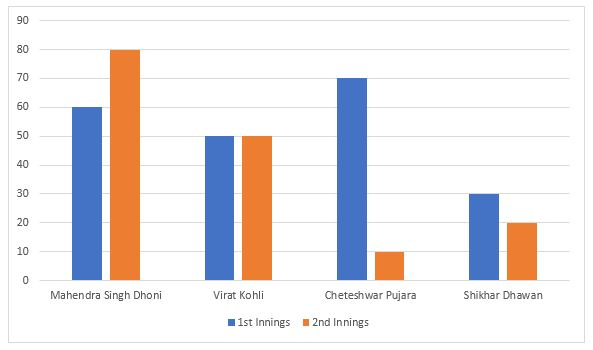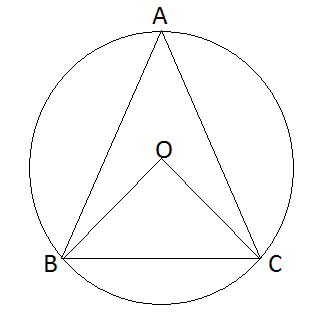# SSC Geometry Questions with Answers

#### Geometry

An excellent collection of SSC Geometry questions and answers with detailed explanations for competitive exams. Given below are some of the most repeated practice questions on Geometry for SSC CGL, CHSL, JE, GD constable, Stenographer, MTS, and CPO exams. Go through this very important online quiz based on model and previous year asked questions from SSC Geometry with solutions to ace the exam.

### Take a free SSC CHSL Mock

Thousands of students have taken Cracku's Free SSC CHSL Mock.

Instructions

For the following questions answer them individually

Question 1

A copper wire is bent in the form of an equilateral triangle, and has an area $$121\sqrt{3}$$ cm. If the same wire is bent into the form of a circle, the area(in cm2) enclosed by the wire in(Take $$\pi = \frac{22}{7}$$)

Instructions

Given here is a multiple bar diagram of the scores of four players in two innings. Study the diagram and answer the questions:Question 2

The total scores in the first innings contributed by the four players is :

Instructions

For the following questions answer them individually

Question 3

What is the area (in sq cm) of a circle whose circumference is 26.4 cm?

Question 4

Find the volume $$(in cm^3)$$ of a sphere of diameter 42 cm.

Question 5

If two medians BE and CF of a triangle ABC, intersect each other at G and if BG = CG, ∠BGC = 60° and BC = 8 cm then area of the triangle ABC is

Question 6

The measures of two angles of a triangle are in the ratio 4 : 5. If the sum of these two measures is equal to the measure of the third angle, find the smallest angle.

Question 7

Two circles touch each other externally. The sum of their areas is 130 $$\pi$$ sq cm and the distance between their centres is 14 cm. The radius of the smaller circle is

Question 8

If D, E and F are the mid points of BC, CA and AB respectively of the AABC then the ratio of area of the parallelogram DEFB and area of the trapezium CAFD is :

Question 9

If the altitude of an equilateral triangle is 12√3 cm, then its area would be :

Question 10

If $$x=\frac{1}{\sqrt{2}+1}$$ then $$(x+1)$$ equals to

Question 11

In triangle ABC, a line is drawn from the vertex A to a point D on BC. If BC = 9 cm and DC = 3 cm, then what is the ratio of the areas of triangle ABD and triangle ADC respectively?

Question 12

Three solid spheres of radius 3 cm, 4 cm and 5 cm are melted and recasted into a solid sphere. What will be the percentage decrease in the surface area?

Question 13

If there are four lines in a plane, then what cannot be the number of points of intersection of these lines?

Question 14

ABC is a right angled triangle in which ∠B = 90°. If BD ⊥ AC, AB = 3 cm and BC = 4 cm, then what is the value of BD (in cm)?

Question 15

The area of a semicircle is $$308 cm^2$$ . Calculate its perimeter (in cm).

Question 16

If ΔFGH is isosceles and FG < 3 cm, GH = 8 cm, then of the following, the true relation is.

Question 17

In Δ ABC, ∠B = 90° and AB : BC = 2 : 1. The value of sin A + cot C is

Question 18

BC is the centre of the circle with centre O. A is a point on major arc BC as shown in the above figure. What is the value of $$\angle{BAC}+\angle{OBC}$$ ?Question 19

The square root of 0.09 is

Question 20

The square root of $$14 + 6\sqrt{5}$$

Question 21

Let $$C_1$$ and $$C_2$$ be the inscribed and circumscribed circles of a triangle with sides 3 cm, 4 cm area of C, and 5 cm then $$\frac{area of C_1}{area of C_2}$$

Question 22

5 persons will live in a tent. If each person requires 16m2 of floor area and 100m3 space for air then the height of the cone of smallest size to accommodate these persons would be

Question 23

The minimum number of common tangents drawn to two circles when both the circles touch each other externally is

Question 24

Two triangles ABC and PQR are congruent. If the area of A ABC is 60 sq. cm, then area of A PQR will be

Question 25

If PQRS is a rhombus and $$\angle$$SPQ = 50°, then $$\angle$$RSQ is

Question 26

If the volume of a sphere is numerically equal to its surface area then its diameter is

Instructions

Given here is a multiple bar diagram of the scores of four players in two innings. Study the diagram and answer the questions:Question 27

The average score in second innings contributed by the four players is :

Instructions

For the following questions answer them individually

Question 28

The percentage increase in the surface area of a cube when each side is doubled is

Question 29

If the length of one side and the diagonal of a rectangle are 8 cm and 17 cm respectively, then find its perimeter (in cm).

Question 30

If the side of a square is reduced by 50%, its area will be reduced by

Question 31

If the opposite sides of a quadrilateral and also its diagonals are equal, then each of the angles of the quadrilateral is

Question 32

The angle subtended by a chord at its centre is 60°, then the ratio between chord and radius is

Question 33

Area of the floor of a cubical room is 48 sq.m. The length of the longest rod that can be kept in that room is

Question 34

If p = 99 then, the value of $$p(p^{2} + 3p + 3)$$ is :

Question 35

Internal bisectors of ∠Q and ∠R of ΔPQR intersect at O. If ∠ROQ = 96° then the value of ∠RPQ is

Question 36

G is the centroid of ΔABC. The medians AD and BE intersect at right angles. If the lengths of AD and BE are 9 cm and 12 cm respectively: then the length of AB (in cm) is

Question 37

If 5 sinθ = 3, the numerical value of $$\frac{\sec\theta-\tan\theta}{\sec\theta+\tan\theta}$$ is

Question 38

The ratio of each interior angle to each exterior angle of a regular polygon is 3 : 1. The number of sides of the polygon is

Question 39

ABC is a cyclic triangle and the bisectors of ∠BAC, ∠ABC and ∠BCA meet the circle at P, Q, and R respectively. Then the angle ∠RQP is

Question 40

For a triangle ABC,D and E are two points on AB and AC such that AD = 1/4 AB, AE =1/4 AC. If BC = 12 cm, then DE is

Question 41

If ABC is an equilateral triangle and P, Q, R respectively denote the middle points of AB, BC, CA then.

Question 42

In a triangle, if orthocentre, circumcentre, incentre and centroid coincide, then the triangle must be

Question 43

If the measure of three angles of a triangle are in the ratio 2 : 3 : 5, then the triangle is :

Question 44

If two supplementary angles differ by 44°, then one of the angles is

Question 45

XY and XZ are tangents to a circle, ST is another tangent to the circle at the point R on the circle, which intersects XY and XZ at S and T respectively. If XY = 15 cm and TX = 9 cm, then RT is

Question 46

In a rhombus ABCD, $$\angle$$A = 60° and AB. = 12 cm. Then the diagonal BD is

Question 47

ABC is a triangle and the sides AB, BC and CA are produced to E, F and G respectively. If $$\angle$$CBE = $$\angle$$ACF = 130° then the value of $$\angle$$GAB is

Question 48

If the sum and difference of two angles are 22/9 radian and 36° respectively, then the value of smaller angle in degree taking the value of $$\pi$$ as 22/7 is :

Question 49

If the three angles of a triangle are : $$(x+15^{\circ})[\frac{6x}{5}+6^{\circ}]$$ and $$[\frac{2x}{3}+30^{\circ}]$$ then the triangle is

Question 50

The length of canvas, 75 cm wide required to build a conical tent of height 14m and the floor area 346.5 m2 is

Question 51

The product of two numbers is 45 and their difference is 4. The sum of squares of the two numbers is

Question 52

AC and BC are two equal cords of a circle. BA is produced to any point P and CP, when joined cuts the circle at T. Then

Question 53

A copper wire is bent in the form of square with an area of 121 cm 2. If the same wire is bent in the form of a circle, the radius (in cm) of the circle is

Question 54

An equilateral triangle of side 6cm has its corners cut off to form a regular hexagon. Area (in cm2) of this regular hexagon will be ?